## Query function with manipulation of dates

This post is in continuation of my earlier post on query function. for basic understand the query function have a look at the following link https://anandexcels.wordpress.com/2013/11/01/query-function-in-google-sheets/

https://anandexcels.wordpress.com/2013/11/07/single-column-manipulation-using-query-function/

https://anandexcels.wordpress.com/2013/11/08/query-function-refering-to-cell-value/

Now we will see how to manipulate Query function with DATES, manipulation of date is query function is tricky. some query does not understand the date because in the spreadsheets dates are treated as serial numbers and query understanding it as text. We have to trick the query function

(Q) Suppose we want list the data based on the certain dates referring to cell

we will continue to use the old example in my first query post

solution is as follows:

=QUERY(A:D;”select * where D>=date”””&text(F1,”yyyy-mm-dd”)&””” and D<=date”””&text(G1,”yyyy-mm-dd”)&””””)

where F1 is the start date and G1 is the end date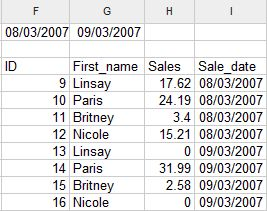Observations

(1) There are three double quote before text function and after text function

(2) we have used the text function to convert the numbers into text

(3) date has to be formatted in yyyy-mm-dd format only.

the link to spreadsheet is available in my first blogpost listed above

## Query function refering to cell value

This post is in continuation of my earlier post on query function. for basic understand the query function have a look at the following link https://anandexcels.wordpress.com/2013/11/01/query-function-in-google-sheets/

Now we look at the referring to cell value within query function so that query function will be more robust.

(Q) What is sales value during the period for Britney

we will continue use our old data set

(A) The formula we are using is

=QUERY(A:D;”select B, Sum(C) where B ='”&F1&”‘ group by B label Sum(C) ‘Sales'”;1)

the results will be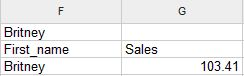where cell F1 contains the criteria in our example Britney

now we do not need to change the formula, if you change the cell value of F1 to from Britney to Paris the query function recalculates the values

the results will be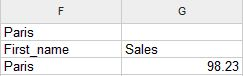Observations:

(1) Remember query function is case sensitive

(2) if you use paris instead of Paris you may get the null string without any error

(3) observe the syntax after where B =

(4) if Cell F1 is string you have use single-quote double-quote ampersand then F1 similarly after F1 also, this is a syntax you have to follow

## Single column manipulation using query function

This post is in continuation of my earlier post on query function. for basic understand the query function have a look at the following link https://anandexcels.wordpress.com/2013/11/01/query-function-in-google-sheets/

we will continue to use my earlier example only.

suppose if we want to count the First name and list them.

we can do this if we have multiple columns of data, suppose we have only one column we want to count as well as list them. you cannot do that using query function alone. we have to manipulate data using the Index function.

the formula for this is

=index(if({1,1},B2:B27))

this will create an array 2 X  26 on fly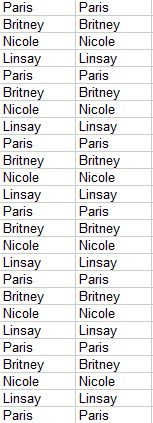Now we have two column of data we can use the query function to manipulate this

=query(index(if({1,1},B2:B27));”select Col1,count(Col2) where Col1 <>” group by Col1 label count(Col2) ‘Count’ “)

the result will be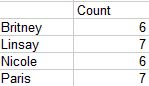Observations:

(1) We have not used the A, B instead we have used Col1 and Col2

(2) because the data is generated using the index function on fly

(3) we can create the copy of existing data using array constants

(4) the logic is within {1,1}

(5) you must use comma (in our example the data is single column), you should not use semi-colon

(6) comma is used for column manipulation and semi-colon is used for row manipulation

(7) this undocumented rules of array

Most versatile effective function and unique to Google Spreadsheets. I am documenting this function to understand it properly and for those who do not have programming background. I do not have previous experience with query. i will try to illustrate with examples.

Google Spreadsheet query is designed to be similar to SQL  with few exceptions. it is a subset of  SQL with a few feature of its own. if you are familiar with SQL it will be easy to learn.

The Syntax of the function is as follow:

DATA: it can be columns(A:C open ranges) of data you want to query, range of cells such as A1:C10, result of function such as importrange, index etc.,

QUERY: It is similar to SQL with small exceptions there is no FROM clause in the this since DATA itself is acting like a FROM clause.

Notes:

1. column headers have to capital letter such A, B, C if you are picking up the raw data with in the same spreadsheet.

2. if you are using the array formulas to manipulate the data (Index, Filter, importrange to name a few) then column headers will Col1, Col2, Col3 etc. observe i have used C capital letter in Col1. this is syntax you have to follow this otherwise you will get a parse error

3. parse error:  an error of language resulting from code that does not conform to the syntax of the programming language; “syntax errors can be recognized at compilation time”

4. Data types: supports data types are string, number, boolean, date, datetime and timeof day. all values of the column will have a data type that matches the column type or a null value

5. The syntax of the query language is composed of the following clauses. Each clause starts with one or two keywords. All clauses are optional. Clauses are separated by spaces. The order of the clauses must be as follows:

 Clause Useage select Select which columns to return, and in what order, if omitted, all the table’s columns are returned, in their default order where Return only rows that match a condition. if omitted, all rows are returned. group by Aggregates values across rows pivot Transforms distinct values in columns into new column order by sorts rows by values in columns limit Limits the number of returned rows offset skips a given number of first rows label sets column labels format formats the values in certain columns using given formatting patterns options sets additional options

now let try to understand these clauses with an example

6. our data set like this

s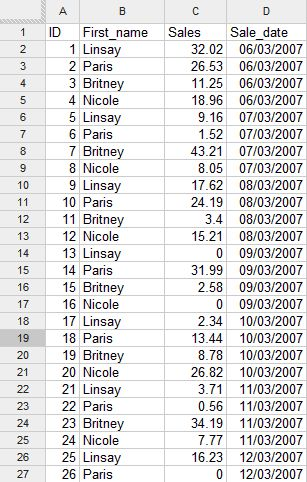7. try this formula

=QUERY(A:D;”select A,B”;1) the result will be as show in the below image

8.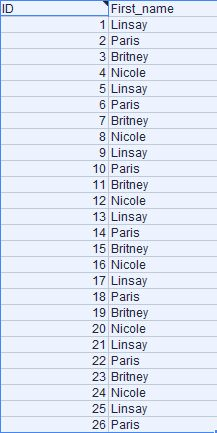We have some special keywords called functions, Functions are bits of code that perform an operation on a value or values. The first we will see is to perform a mathematical operation on a column. We will see Sum function which by totaling the values in a column designated by parentheses.

suppose we want to Sum Column C where the Column B is Nicole

=QUERY(A:D;”select B, Sum(C) where B = ‘Nicole’ group by B”;1)

9. the result will be

10.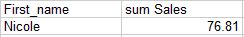Note:

(1) you have to use S capital in the Sum followed by column you want sum in parentheses

(2) whenever you are using the group by clause same column has to be selected in the select clause otherwise you may get value error

(3) condition you want the check in the column B has to be in single quote

this can be sorted using the order by clause

=QUERY(A:D;”select B, Sum(C) where B <>” group by B order by B asc”;1)

the result will be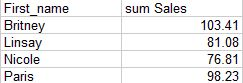Note:

(1) observe the column 1 has been sorted in ascending order

(2) to get the the descending order you can use the desc instead of asc

11. you can also sort the based on the result of the Sum(C)

=QUERY(A:D;”select B, Sum(C) where B <>” group by B order by Sum(C) desc”;1)

the results will be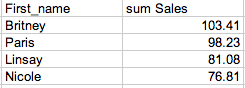Note:

(1) we have sorted data by descending order based on Sum of sales

(2) you have to follow the order of clause listed above

We can also limit the results top 3 or top 2 using the limit clause

=QUERY(A:D;”select B, Sum(C) where B <>” group by B order by Sum(C) desc limit 3″;1)We can also the name the column sum Sales as Top 3 sales

=QUERY(A:D;”select B, Sum(C) where B <>” group by B order by Sum(C) desc limit 3 label Sum(C) ‘Top 3 Sales'”;1)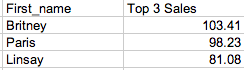We can also pivot the data based on date in the D column.

=QUERY(A:D;”select B, Sum(C) where B <>” group by B pivot D”;1)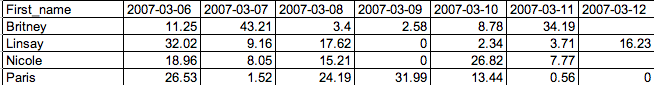Note:

(1) You might have observed the column D is not selected the select clause

(2) Date are formatted in yyyy-mm-dd format

(3) pivot is unique to google Sheets Query function

12. In the next blog post we will see how to manipulate data using the cell value